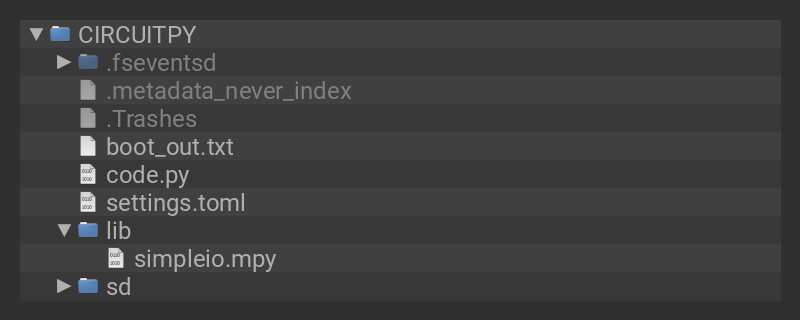The Arduino Nano RP2040 Connect has a PDM microphone onboard, the MP34DT05. It can be controlled in CircuitPython with `board.MICROPHONE_CLOCK` and `board.MICROPHONE_DATA` with the `audiobusio` library. The example code below has been modified from the PDM Microphone Breakout Learn Guide. Here the PDM's input data is visualized in two ways. First, it is plotted to the serial plotter in Mu. Secondly, it's sent to an LED via PWM.

## Wiring

• LED's anode to pin A1 with a 220 Ω resistor
• LED's cathode to GND

In the example below, click the Download Project Bundle button below to download the necessary libraries and the code.py file in a zip file. Extract the contents of the zip file, open the directory Arduino_Nano_RP2040_Connect/arduino_nano_rp2040_connect_mic/ and then click on the directory that matches the version of CircuitPython you're using and copy the contents of that directory to your CIRCUITPY drive.

Your CIRCUITPY drive should now look similar to the following image:```# SPDX-FileCopyrightText: 2021 Liz Clark for Adafruit Industries
#

'''Adapted from the example code DM_Mic_Sound_Level_Plot.py
blob/master/PDM_Microphone/PDM_Mic_Sound_Level_Plot.py '''

import time
import array
import math
import board
import audiobusio
import pwmio
import simpleio

# LED setup
led = pwmio.PWMOut(board.A1, frequency=5000, duty_cycle=0)

# Remove DC bias before computing RMS.
def mean(values):
return sum(values) / len(values)

def normalized_rms(values):
minbuf = int(mean(values))
samples_sum = sum(
float(sample - minbuf) * (sample - minbuf)
for sample in values
)

return math.sqrt(samples_sum / len(values))

mic = audiobusio.PDMIn(board.MICROPHONE_CLOCK,
board.MICROPHONE_DATA, sample_rate=16000, bit_depth=16)
samples = array.array('H',  * 160)

while True:

mic.record(samples, len(samples))
magnitude = normalized_rms(samples)
print((magnitude,))

#  mapping mic's level to LED PWM range
mapped_value = simpleio.map_range(magnitude, 75 , 300, 0, 65535)

#  sending mapped value to LED for PWM
led.duty_cycle = int(mapped_value)
#  optional logging for mapped_value
#  print((mapped_value,))
time.sleep(0.01)
```

## Plotting Data

To view the plotter in Mu, click on the Plotter icon at the top of the Mu window.

This will open a plotter window at the bottom of your Mu window. As the microphone brings in data, you'll see it plot in real-time.

## Mic Data as PWM

First, `led` is setup as a PWM output.

```# LED setup
led = pwmio.PWMOut(board.A1, frequency=5000, duty_cycle=0)```

Then, in the loop, `map_range` is used to map `magnitude`'s range of `75` to `300` to the PWM range of `0` to `65535`. `magnitude` is the variable that is holding the mic's incoming data. Starting the range at `75` for `magnitude` allows for the filtering out of background noise.

```#  mapping mic's level to LED PWM range
mapped_value = simpleio.map_range(magnitude, 75 , 300, 0, 65535)```

Finally, `mapped_value` is sent as an integer to the `led`'s `duty_cycle` for PWM.

```#  sending mapped value to LED for PWM
led.duty_cycle = int(mapped_value)```

Your LED's brightness will change depending on the volume of sound being heard by the PDM mic.

This guide was first published on Jun 04, 2021. It was last updated on Jun 04, 2021.